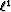Next: Introduction Up: Adaptive subtraction of multiples Previous: Adaptive subtraction of multiples

# Summary

A strategy for multiple removal consists in estimating a model of the multiples and then adaptively subtracting this model to the data by estimating shaping filters. A possible and efficient way of computing these filters is by minimizing the difference or misfit between the input data and the filtered multiples in a least-squares sense. Therefore, the signal is assumed to have minimum energy and to be orthogonal to the noise. Some problems arise when these conditions are not met. For instance, for strong primaries with weak multiples, the multiple model might be matched to the signal (primaries) and not to the noise (multiples). Consequently, when the signal does not exhibit minimum energy, I propose using thenorm as opposed to thenorm for the filter estimation step. This choice comes from the well-known fact that thenorm is robust to large'' amplitude differences when measuring data misfit. Thenorm is approximated with the Huber norm (Chapter) minimized with a quasi-Newton method. This technique is an excellent approximation to thenorm. I illustrate this method with synthetic and field data where internal multiples are attenuated. I show that thenorm leads to a much improved attenuation of the multiples when the minimum energy assumption is violated. In particular, the multiple model is fitted to the multiples in the data only, while preserving the primaries.Next: Introduction Up: Adaptive subtraction of multiples Previous: Adaptive subtraction of multiples
Stanford Exploration Project
5/5/2005